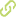# Cosine policy iteration for solving infinite-horizon markov decision processes Chapter in Scopus•
• Overview
•
• Identity
•
•
• View All
•

### Abstract

• Police Iteration (PI) is a widely used traditional method for solving Markov Decision Processes (MDPs). In this paper, the cosine policy iteration (CPI) method for solving complex problems formulated as infinite-horizon MDPs is proposed. CPI combines the advantages of two methods: i) Cosine Simplex Method (CSM) which is based on the Karush, Kuhn, and Tucker (KKT) optimality conditions and finds rapidly an initial policy close to the optimal solution and ii) PI which is able to achieve the global optimum. In order to apply CSM to this kind of problems, a well- known LP formulation is applied and particular features are derived in this paper. Obtained results show that the application of CPI solves MDPs in a lower number of iterations that the traditional PI. © 2009 Springer-Verlag Berlin Heidelberg.

### Publication date

• December 2, 2009